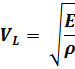The velocity of a longitudinal acoustic wave in a solid material depends on the nature of the material and can be calculated by the next formula:

Formula:## Where:

V is the velocity of the longitudinal wave in meter per second (m/s)

E is the Young´s Modulus (*)

ρ is the desnsity

(*) Young´s Modulus is numerically equal to the stress required to double the length of a body. Actually, in most bodies, rupture occurs at considerable smaller stresses.